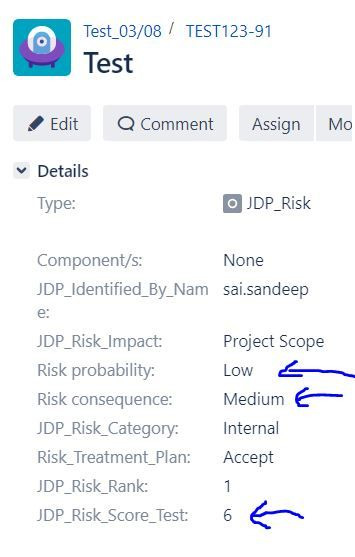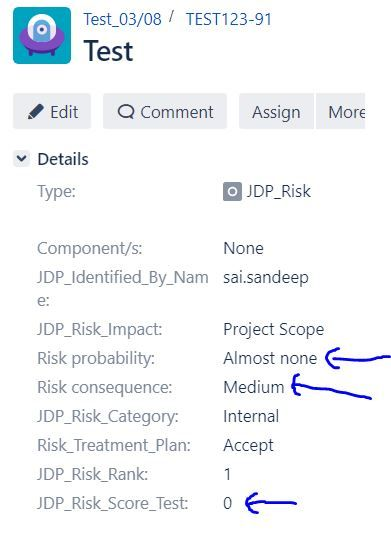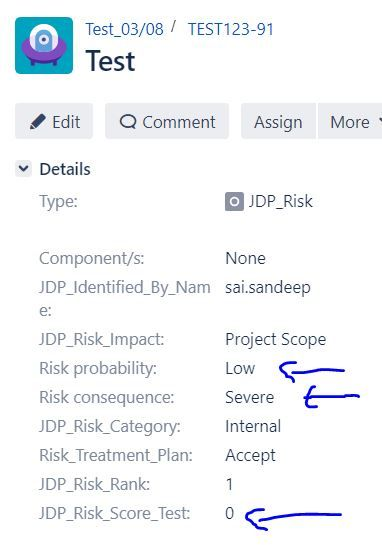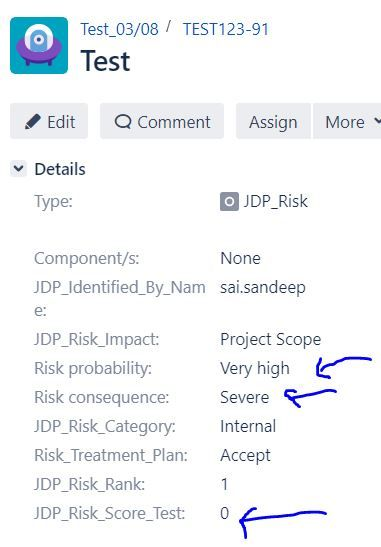cancel
Showing results for
Did you mean:
See all
See all
##### Top groups
Explore all groups

## Come for the products,stay for the community

The Atlassian Community can help you and your team get more value out of Atlassian products and practices.4,364,839
Community Members

Community Events
168
Community Groups

# My code is not working properly in Script Runner for few of the switch cases.

Hi,

I have developed a custom scripted field for calculating JDP Risk Score using Script Runner Tool. We have also written the code for the field but code is not working completely.

I am attaching the code here.

import com.atlassian.jira.component.ComponentAccessor
import com.atlassian.jira.issue.CustomFieldManager
import com.atlassian.jira.issue.Issue
import com.atlassian.jira.issue.fields.CustomField
import org.apache.log4j.Logger

/**
* Script which reads text value from two custom fields and converts then to a numeric value.
* By default, two custom fields which are taken into consideration:
* - 'Risk probability'
* - 'Risk consequence'
*
* For each field a set of possible values is defined in this script.
* Each value is assigned a numerical value which is later used for multiplication.
* Assignment of text to a number is done in 'switch' blocks.
* To map a new text value to a number, insert 3 lines:
* case "Input the text value to map":
* consequenceNumberValue = "Input the number to be mapped as a n integer e.g. 5";
* break;
* Example of such new mapping for Risk consequence:
* case "Critical":
* consequenceNumberValue = 9;
* break;
* Example for new Risk probability mapping:
* case "Very low":
* probabilityNumberValue = 1;
* break;
*
* Script can be extended to calculate the Ranking with different formula and using different amount of data.
* */

def log = Logger.getLogger("scripts.risk.RiskRankingFieldFormula")
Issue issue = issue;
String riskProbabilityName = "Risk probability";
String riskConsequenceName = "Risk consequence";
CustomFieldManager customFieldManager = ComponentAccessor.getCustomFieldManager();
try {
List<CustomField> listOfConsequenceCustomFields = customFieldManager.getCustomFieldObjectsByName(riskConsequenceName);
CustomField riskCustomField;
if (listOfConsequenceCustomFields.isEmpty()) {
throw new Exception("Could not find a custom field with name '" + riskConsequenceName + "'");
} else {
riskCustomField = listOfConsequenceCustomFields.get(0);
}

String consequenceTextValue = issue.getCustomFieldValue(riskCustomField);
Integer consequenceNumberValue;

switch (consequenceTextValue) {
/** Insert new mappings after this line*/
case "Almost none":
consequenceNumberValue = 1;
break;
case "Low":
consequenceNumberValue = 2;
break;
case "Medium":
consequenceNumberValue = 3;
break;
case "High":
consequenceNumberValue = 4;
break;
case "Very high":
consequenceNumberValue = 5;
break;
default: //Setting the value to zero will force the multiplication
consequenceNumberValue = 0;
}

List<CustomField> listOfProbabilityCustomFields = customFieldManager.getCustomFieldObjectsByName(riskProbabilityName);
CustomField probabilityCustomField;
if (listOfProbabilityCustomFields.isEmpty()) {
throw new Exception("Could not find a custom field with name '" + riskProbabilityName + "'");

} else {
probabilityCustomField = listOfProbabilityCustomFields.get(0)
}
String probabilityTextValue = issue.getCustomFieldValue(probabilityCustomField)
Integer probabilityNumberValue;
switch (probabilityTextValue) {
/** Insert new mappings after this line*/
case "Trivial":
probabilityNumberValue = 1;
break;
case "Low":
probabilityNumberValue = 2;
break;
case "Medium":
probabilityNumberValue = 3;
break;
case "High":
probabilityNumberValue = 4;
break;
case "Severe":
probabilityNumberValue = 5;
break;
default:
probabilityNumberValue = 0;
}
/** Multiply both numbers values to receive a final Risk ranking as an integer*/
Integer calculatedRiskRanking = probabilityNumberValue * consequenceNumberValue;
return calculatedRiskRanking;

} catch (Exception e) {
log.error("Caught exception: " + e);
// e.printStackTrace()
/** Return -1 to indicate that something did not go as planning in the algorithm*/
return -1;
}

In this code, the Risk Score is not getting calculated for switch case of 'Almost none' and 'Very high' in Risk Consequence and for switch case of "Trivial' and 'Severe' in Risk Probability.

For switch cases of 'Low', 'Medium' and 'High', the code is working fine.

I am attaching the snapshot of error. Please guide us accordingly.